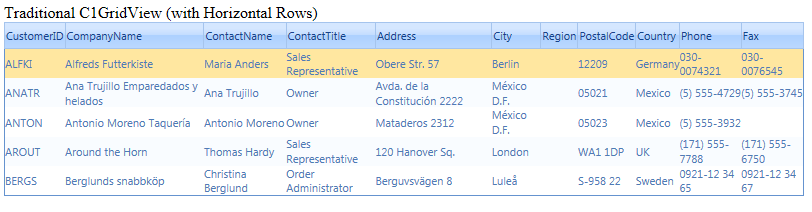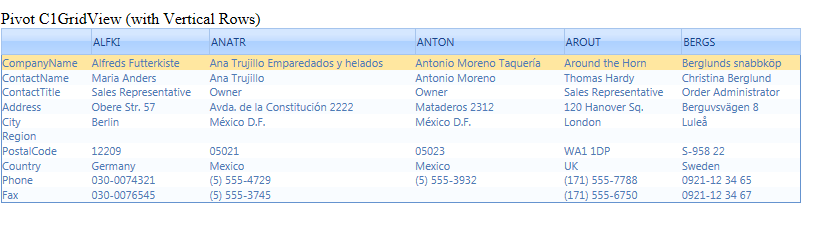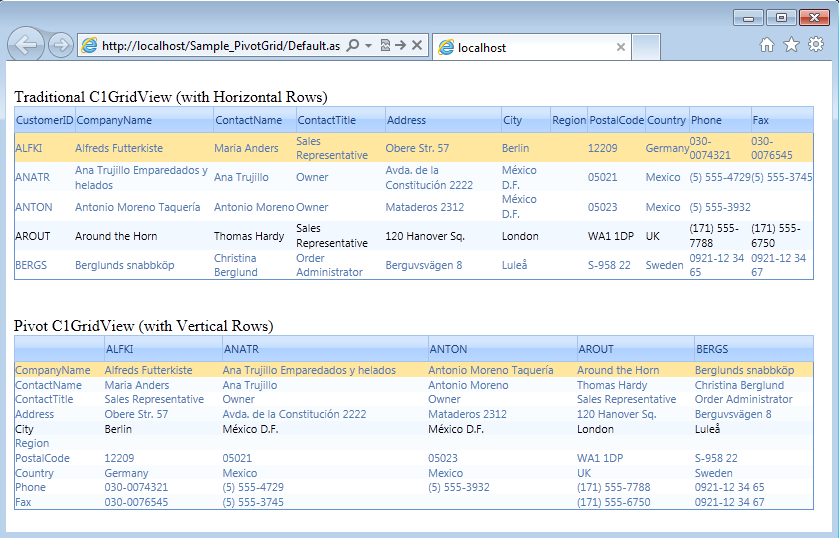The C1GridView displays the grid as a table. When a user wants to display grid in a horizontal manner (vertical rows), there is no property in the grid to flip the grid. This blog provides an easy way of displaying the grid as pivot grid, that is display grid with vertical rows. Traditionally, the C1GridView displays data as :With this blog we aim to display the grid with vertical rows:We will create a method 'FlipDataSet()' which will flip all the tables. That is, rows will become columns and columns will become rows :

``````//Flip DataSet
public DataSet FlipDataSet(DataSet my_DataSet)
{
DataSet ds = new DataSet();
foreach (DataTable dt in my_DataSet.Tables)
{
DataTable table = new DataTable();

foreach (DataRow r in dt.Rows)

for (int i = 0; i < dt.Columns.Count - 1; i++)
{
}

for (int r = 0; r < table.Rows.Count; r++)
{
for (int c = 0; c < table.Columns.Count; c++)
{
if (c == 0)
table.Rows[r] = dt.Columns[r + 1].ColumnName;
else
table.Rows[r][c] = dt.Rows[c - 1][r + 1];
}
}
table.AcceptChanges();

The following image shows the difference between grids with horizontal and vertical rows.Please download the Sample_PivotGrid for the above implementation.